# RD Sharma Solutions for Class 9 Maths Chapter 8 Lines and Angles Exercise 8.3

RD Sharma Class 9 Maths solutions chapter 8 exercise 8.3 is available here. All exercise questions are solved by subject experts at BYJU’S using a step-by-step problem-solving approach. RD Sharma Solutions class 9 exercise 8.3 is based on some useful relations on pair of angles, especially vertically opposite angles.

## Download PDF of RD Sharma Solutions for Class 9 Maths Chapter 8 Lines and Angles Exercise 8.3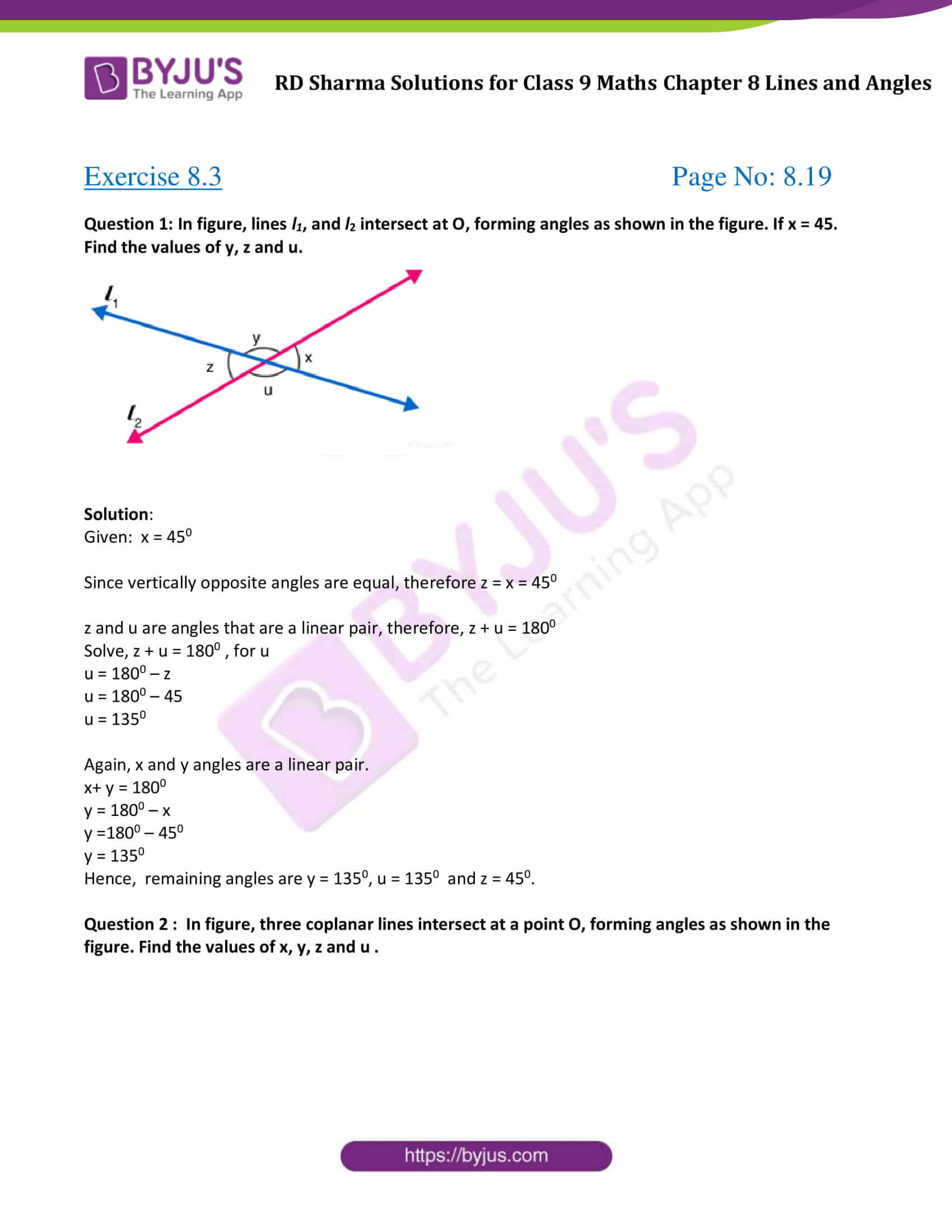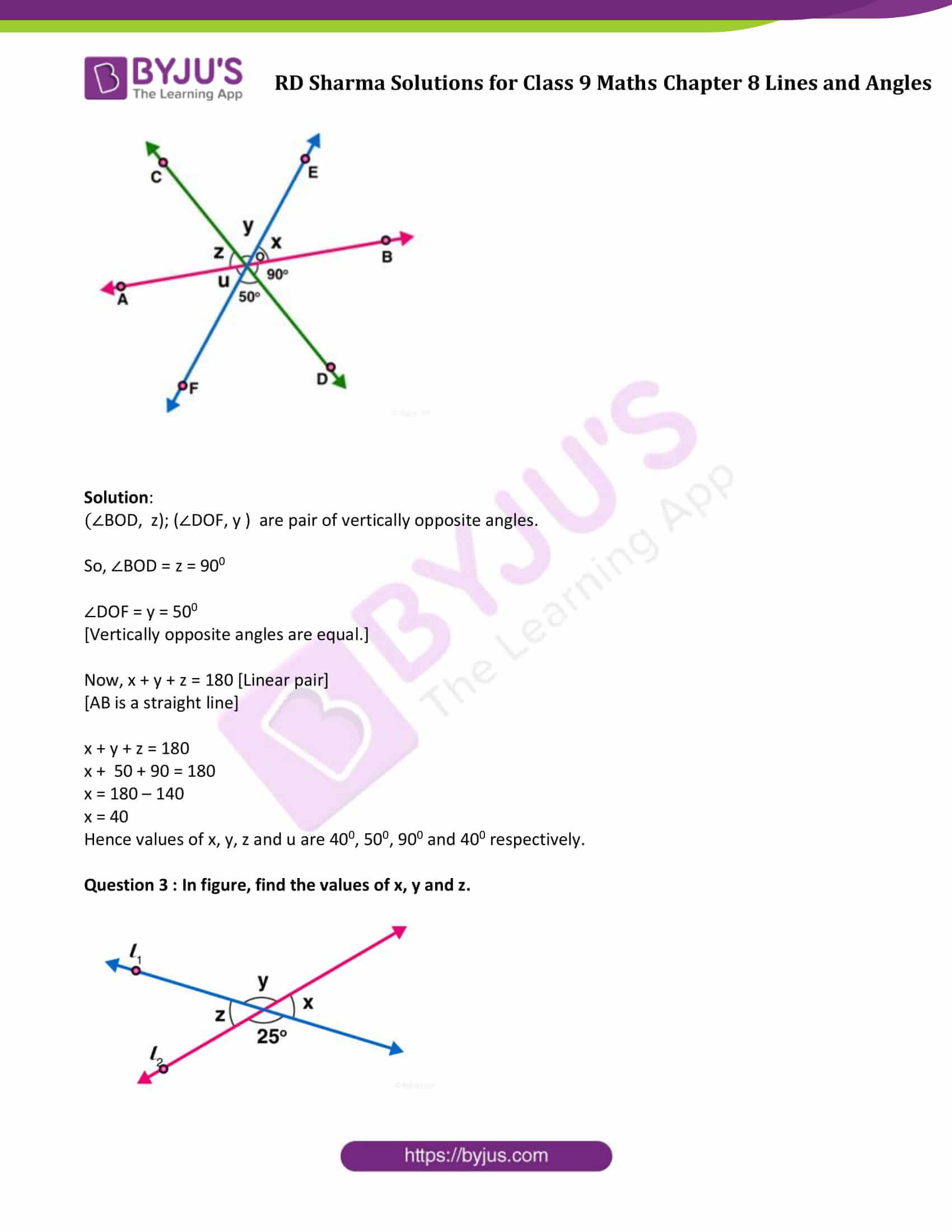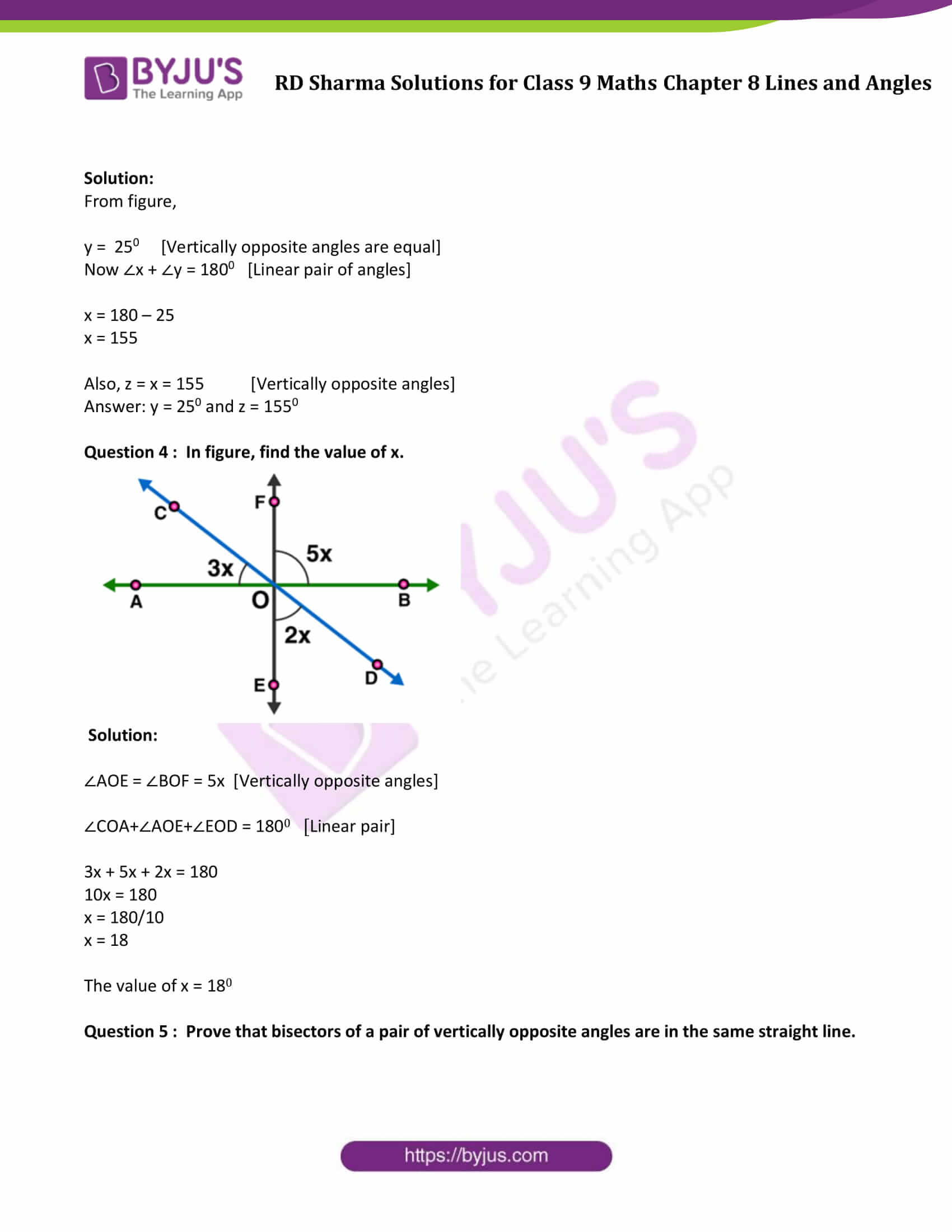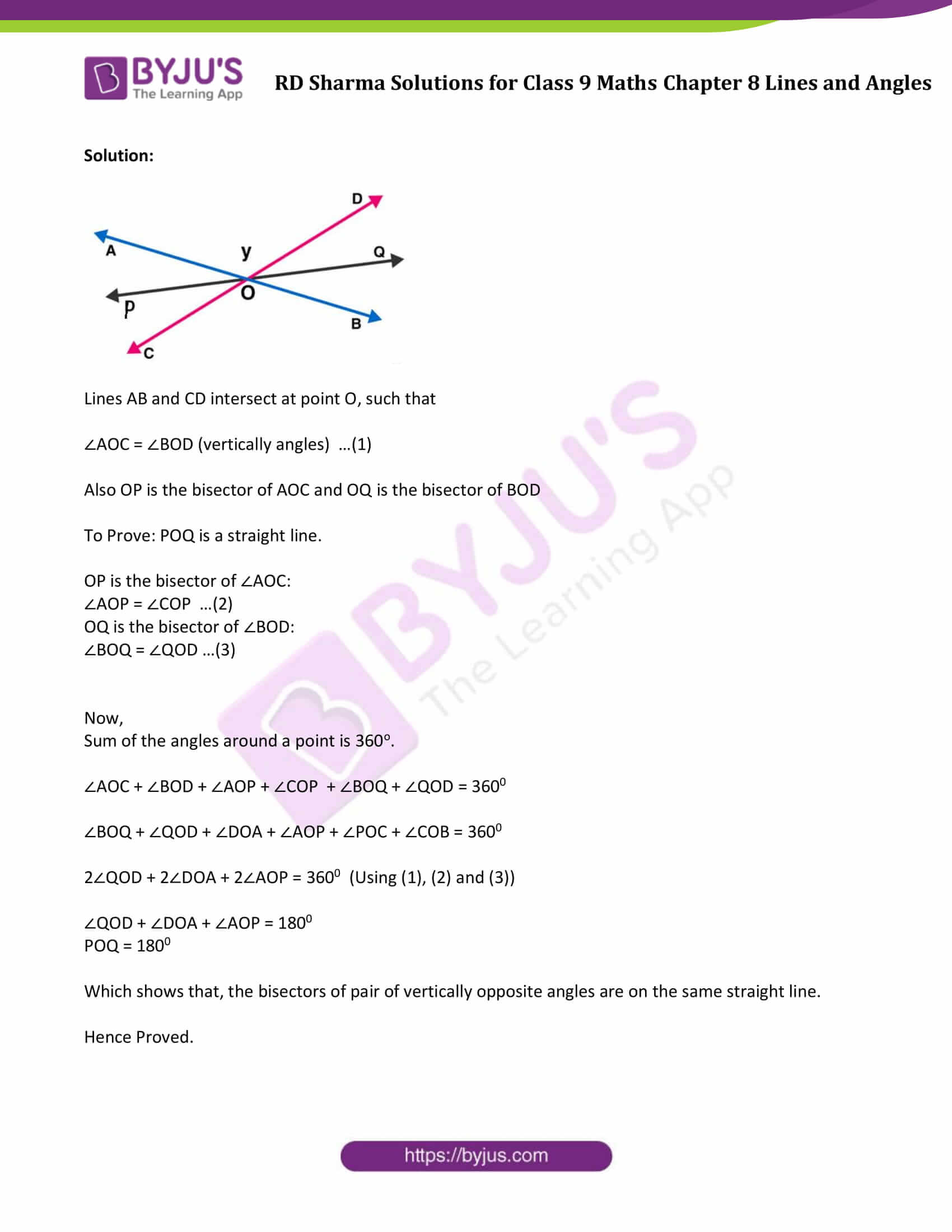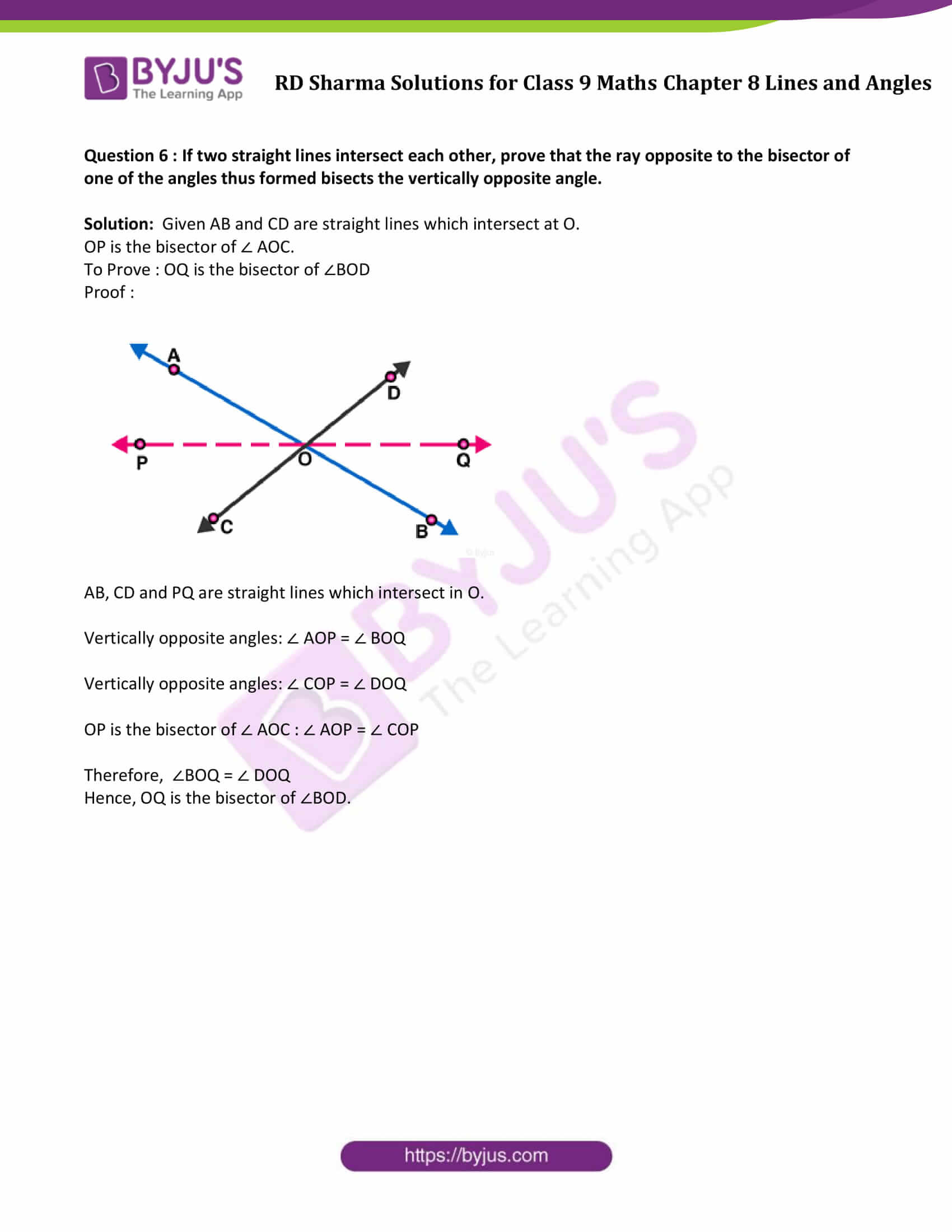### Access Answers to Maths RD Sharma Solutions for Class 9 Chapter 8 Lines and Angles Exercise 8.3 Page number 8.19

Question 1: In figure, lines l1, and l2 intersect at O, forming angles as shown in the figure. If x = 45. Find the values of y, z and u.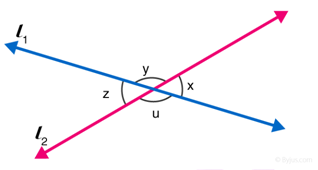Solution:

Given: x = 450

Since vertically opposite angles are equal, therefore z = x = 450

z and u are angles that are a linear pair, therefore, z + u = 1800

Solve, z + u = 1800 , for u

u = 1800 – z

u = 1800 – 45

u = 1350

Again, x and y angles are a linear pair.

x+ y = 1800

y = 1800 – x

y =1800 – 450

y = 1350

Hence, remaining angles are y = 1350, u = 1350 and z = 450.

Question 2 : In figure, three coplanar lines intersect at a point O, forming angles as shown in the figure. Find the values of x, y, z and u .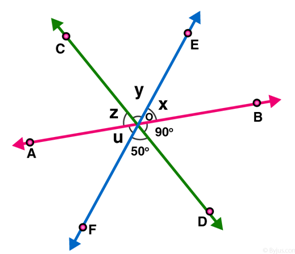Solution:

(∠BOD, z); (∠DOF, y ) are pair of vertically opposite angles.

So, ∠BOD = z = 900

∠DOF = y = 500

[Vertically opposite angles are equal.]

Now, x + y + z = 180 [Linear pair] [AB is a straight line]

x + y + z = 180

x + 50 + 90 = 180

x = 180 – 140

x = 40

Hence values of x, y, z and u are 400, 500, 900 and 400 respectively.

Question 3 : In figure, find the values of x, y and z.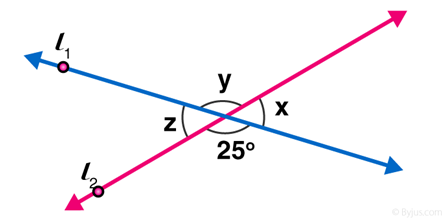Solution:

From figure,

y = 250 [Vertically opposite angles are equal]

Now ∠x + ∠y = 1800 [Linear pair of angles]

x = 180 – 25

x = 155

Also, z = x = 155 [Vertically opposite angles]

Answer: y = 250 and z = 1550

Question 4 : In figure, find the value of x.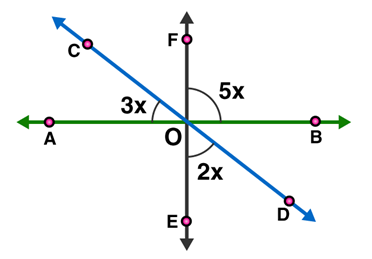Solution:

∠AOE = ∠BOF = 5x [Vertically opposite angles]

∠COA+∠AOE+∠EOD = 1800 [Linear pair]

3x + 5x + 2x = 180

10x = 180

x = 180/10

x = 18

The value of x = 180

Question 5 : Prove that bisectors of a pair of vertically opposite angles are in the same straight line.

Solution: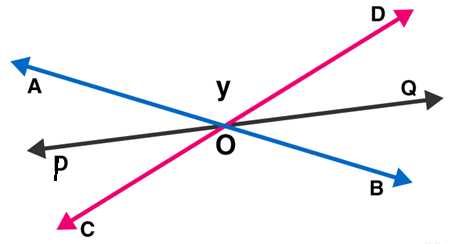Lines AB and CD intersect at point O, such that

∠AOC = ∠BOD (vertically angles) …(1)

Also OP is the bisector of AOC and OQ is the bisector of BOD

To Prove: POQ is a straight line.

OP is the bisector of ∠AOC:

∠AOP = ∠COP …(2)

OQ is the bisector of ∠BOD:

∠BOQ = ∠QOD …(3)

Now,

Sum of the angles around a point is 360o.

∠AOC + ∠BOD + ∠AOP + ∠COP + ∠BOQ + ∠QOD = 3600

∠BOQ + ∠QOD + ∠DOA + ∠AOP + ∠POC + ∠COB = 3600

2∠QOD + 2∠DOA + 2∠AOP = 3600 (Using (1), (2) and (3))

∠QOD + ∠DOA + ∠AOP = 1800

POQ = 1800

Which shows that, the bisectors of pair of vertically opposite angles are on the same straight line.

Hence Proved.

Question 6 : If two straight lines intersect each other, prove that the ray opposite to the bisector of one of the angles thus formed bisects the vertically opposite angle.

Solution: Given AB and CD are straight lines which intersect at O.

OP is the bisector of ∠ AOC.

To Prove : OQ is the bisector of ∠BOD

Proof :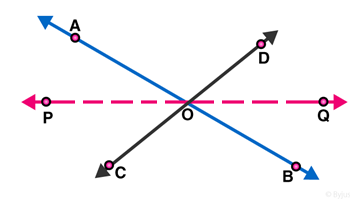AB, CD and PQ are straight lines which intersect in O.

Vertically opposite angles: ∠ AOP = ∠ BOQ

Vertically opposite angles: ∠ COP = ∠ DOQ

OP is the bisector of ∠ AOC : ∠ AOP = ∠ COP

Therefore,  ∠BOQ = ∠ DOQ

Hence, OQ is the bisector of ∠BOD.

## RD Sharma Solutions for Class 9 Maths Chapter 8 Lines and Angles Exercise 8.3

Class 9 Maths Chapter 8 Lines and Angles Exercise 8.3 is based on vertically opposite angles. Students can freely access RD Sharma Solutions chapter 8 exercise solutions and prepare for their exams.